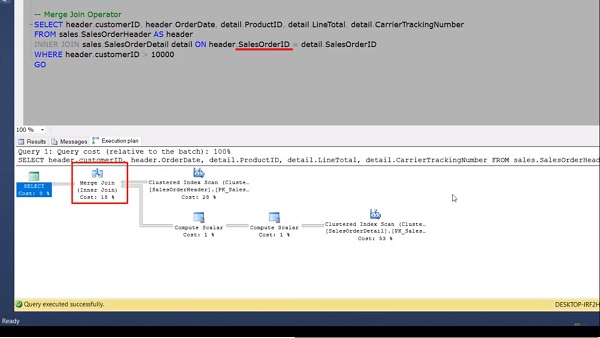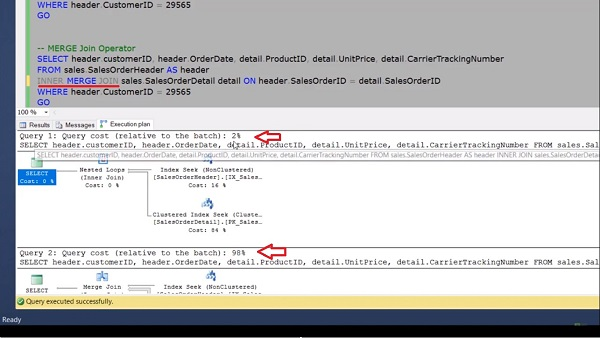# TSQL Execution Plan Operator - Merge Join

SQL Server employs four types of physical join operations to carry out the logical join operations. Merge Join is one of the physical operators that SQL server uses in full join, left join, or inner join and provides an output that is generated by joining two sorted data sets.

If your input tables are large then merge join is the fastest join operation but the merge join requires both input tables to be sorted on the joining columns, which are defined by the “ON” clauses of the join predicate. In this video, we’ll be going to learn about this physical operator.

In this session, we’ll be going to learn about one of the T-SQL execution plan operators called Merge Join. The Merge Join is one of the physical operators that SQL Server uses in Full join, Left join, or Inner join and provides an output that is generated by joining two sorted data sets. If your input table is large, then Merge Join is the fastest join operation. But the Merge Join requires both input tables to be shorted on the joining columns, which are defined by the ON clause of the join predicate.

When each input is sorted, the Merge Join operator gets a row from the outer input table and compares them with the inner input table. If the matching row is found, then return the records. When no matching row is found, then obtain a new row from the smaller input table. This process repeats until all rows have been processed.

Here for this demo, we will again use the same query which we have used in the previous session of Nested Loop. But here with a minor change in the where clause. Now, enable the execution plan and execute the query. If we look at the execution plan, we can see that our query is using a Merge Join. In this case, the query optimizer decides to use a Merge Join, as both input tables are large in terms of rows and they are also pre-indexed or shorted on this (Sales Order ID) column.If I hover over the outer input table, which is the sales order header, we can see that the actual number of rows is 31465. When I hover over the input table, then here the actual number of rows is 121317. Now if we go into the message tab, we can see that both input tables are scanned only once as opposed to the eight scans, which we have already shown in the previous demo of Nested Loop Join.

In the execution plan, we can see the Compute Scalar operator because we are selecting the line total column in the select statement, which is a derived column. Hence this operator is being used in the execution plan. Now, if we go into the previous session query of nested loop and try to force the Merge Join

by writing a merge forcefully then after executing both queries, we can see the cost relative to this page for this query is only 2%.For the Merge Join, the cost of the same query relative to this page is 98%. So don’t force the Merge Join until and unless it is required in the specific scenario.